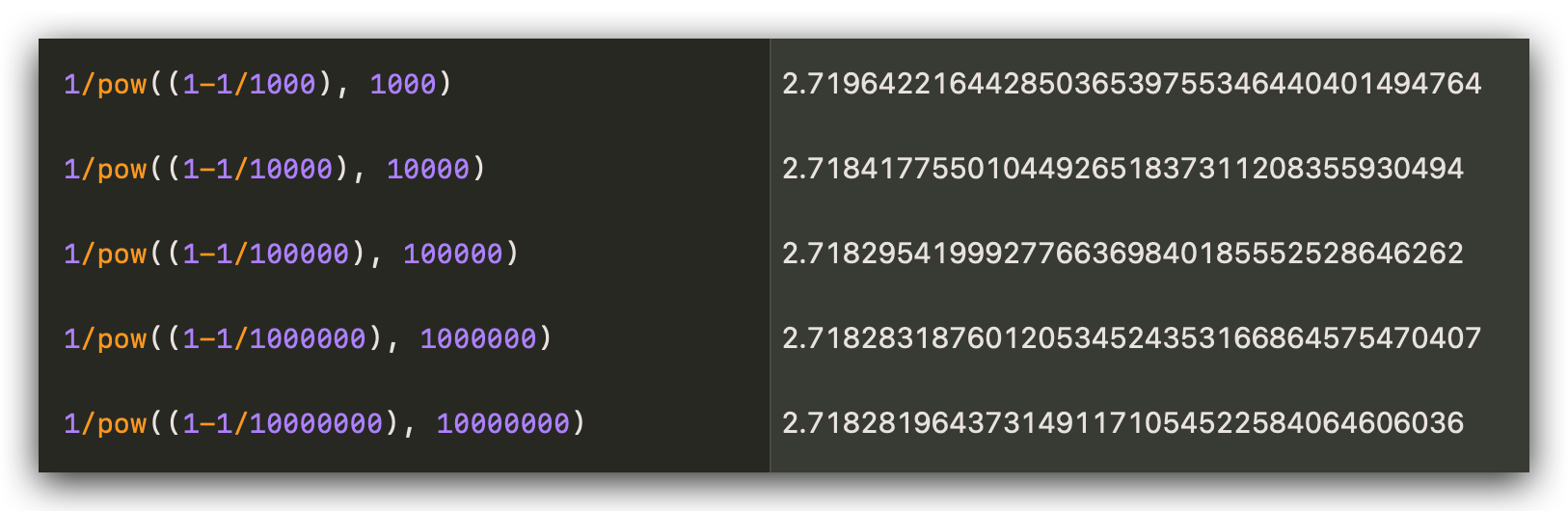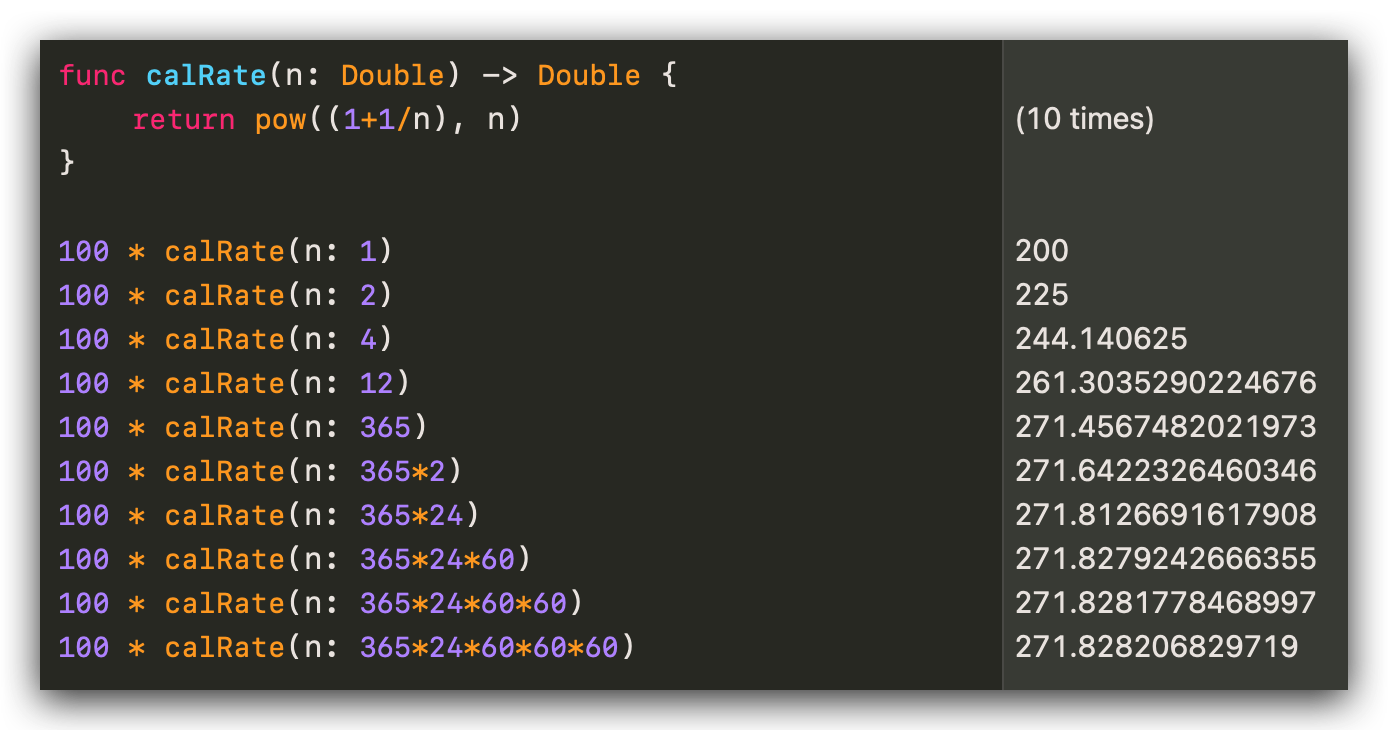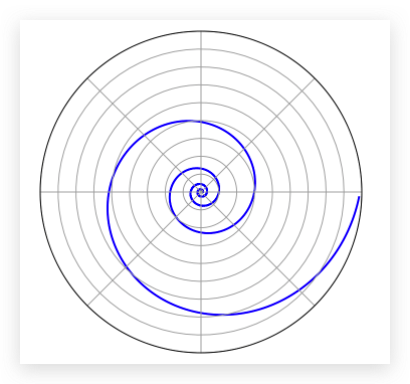# 自然常数 e 为什么冠以“自然”二字

$\pi$ 应该是我们最熟悉的数学常数了，但是除了 $\pi$ 以外，还有一个数学常数 $e$，它同样重要，同样与我们生活息息相关，甚至被冠名为 自然常数，它是对数函数的底数，有时候也称它为欧拉数（Euler number），以瑞士数学家欧拉命名。

$$e = \lim\limits_{n \to \infty} (1+\frac{1}{n})^n$$$$1-\frac{1}{1000}$$

$$(1-\frac{1}{1000})^{1000} \approx 0.36769542477096404462680613922046134399$$

$$\frac{1}{0.367695424770964044626..} \approx 2.71964221644285036539755346440401494764$$

$$(1-\frac{1}{10000})^{10000} \approx 0.3678610464329299241113356722185087445$$

$$\frac{1}{0.3678610464329299241113356722185087445} \approx 2.7184177550104492651837311208355930494$$

$$\frac{1}{(1-\frac{1}{n})^n}$$$$\lim\limits_{n \to \infty} (1-\frac{1}{n})^n = \frac{1}{e}$$

$$(1-\frac{1}{1000})^{1000} \approx 0.368$$

$$1000 \times 0.368 = 368$$

$$100 \times (1 + 100\%) = 200$$

$$100 \times (1+\frac{100\%}{2}） \times (1+\frac{100\%}{2}) = 225$$

$$100 \times (1+\frac{100\%}{4})^4 \approx 244$$$$\lim\limits_{n \to \infty} (1+\frac{1}{n})^n = e$$$$r = ae^{b\theta}$$